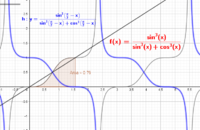# Surprising result?

#### Dr.Peterson

##### Elite Member
It looks to me like a matter of symmetry; the curve under which you are integrating is symmetrical about the point (pi/4, 1/2).

The area in each case is half of a rectangle with base pi/2 and height 1, because the curve bisects that rectangle. And that happens because f(pi/2 - x) = 1 - f(x).

•topsquark

#### Dr.Peterson

##### Elite Member
Yes, certain definite integrals are much easier than their indefinite integrals ...

I saw the answer purely visually; the last graph was most obvious, then I saw the symmetry in the others, and then I realized how to see that in the function itself. It's all about cofunctions.

#### apple2357

##### Full Member
I can see your transformations explanation works, i can't quite see why f(pi/2-x) is the same as f(x) but reflected in the way it is ( as below)
If it was f(x-pi/2) i would understand that as a simple translation but is f(pi/2-x) is a combination of transformations? Is this a translation and a reflection?How did you come up with f(pi/2-x) = 1-f(x) as the explanation for the graphical observation?

#### Dr.Peterson

##### Elite Member
I'd call it a double reflection, first around x=pi/4 and then around y=1/2. Equivalently, and the way I described it initially, it is actually a rotation around the point (pi/4, 1/2). In the same way, reflecting in both y=0 and x=0 amounts to rotating by 180 degrees about the origin.

Replacing x with pi/2 - x reflects in x=pi/4, and replacing y with 1-y (that is, subtracting the result from 1) reflects in y=1/2. These are worth pondering until you see why!

#### apple2357

##### Full Member
I'd call it a double reflection, first around x=pi/4 and then around y=1/2. Equivalently, and the way I described it initially, it is actually a rotation around the point (pi/4, 1/2). In the same way, reflecting in both y=0 and x=0 amounts to rotating by 180 degrees about the origin.

Replacing x with pi/2 - x reflects in x=pi/4, and replacing y with 1-y (that is, subtracting the result from 1) reflects in y=1/2. These are worth pondering until you see why!
So If we reflect y=f(x) in x=a, we get the curve y=f(−x+2a) ?

Is this because if you want to move every point to a position the same distance the other side of x=a we end up at x+2(a-x). Which gives us 2a -x
Is that how you would explain it?

#### Dr.Peterson

##### Elite Member
Yes, that is one good way to explain this fact.

And, of course, the same fact is why the other reflection is 1 - y.